• Call Now

1800-102-2727•

# Vector Addition: Laws of Vector Addition, Properties, Practice Problems and FAQs

Suppose you are playing a special type of game in which a rope is tightened on your waist and two of your friends are pulling you, one towards east and another towards north. But you will feel that you are not going toward any of those directions and going between them.

Table of Content

## Angle Between Two Vectors

Angle between two vectors means angle between directions of these vectors when they are placed tail to tail by displacing either of the vectors.

Let two vector A and B and angle between them is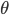then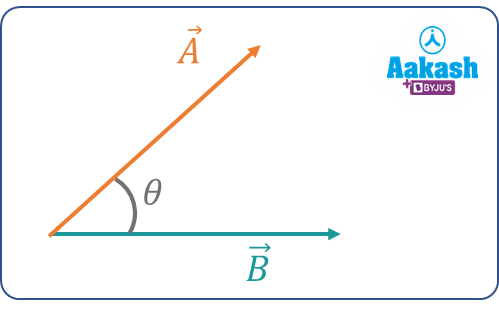Now let two vectors C and D After shifting vector C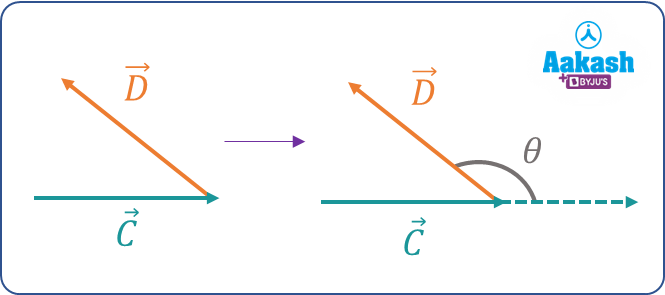Vector addition is the operation of adding two or more vectors together to find a resultant vector.

There are many ways to vector addition.

Vectors can be added graphically according to three laws which are as follows:

• Triangle law of vectors addition
• Parallelogram law of vectors addition
• Polygon law of vectors addition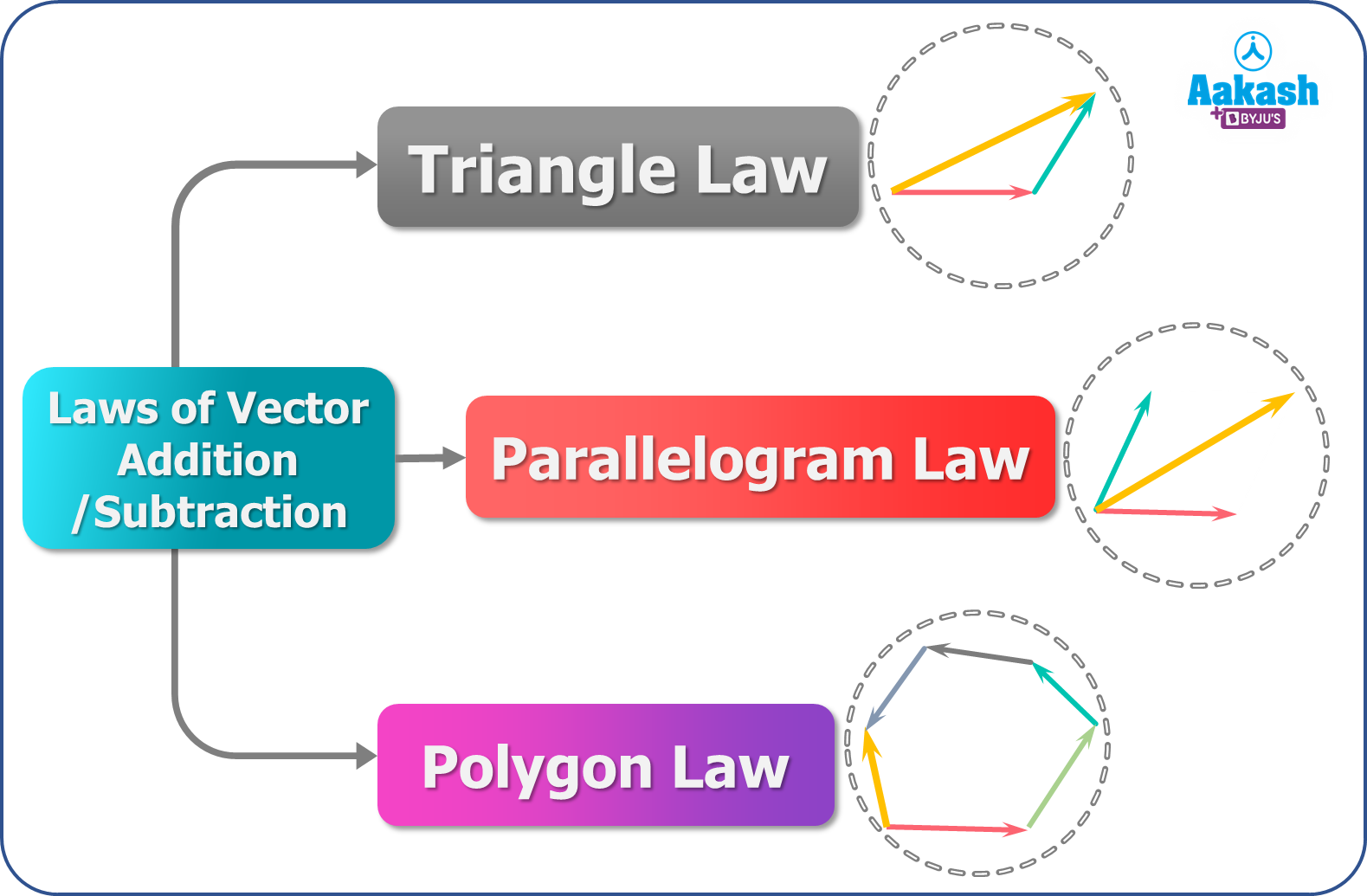## Triangle Law of Vector Addition

In the triangle law of vector addition, When the magnitude and direction of two vectors are represented by the two sides of a triangle such that the tail of the second vector is at the head of first vector then the third side will represent the resultant of vector and direction will be form tail of first vector to head of second vector .

Let A and B be the given vector, then the resultant of these two vectors R=A+B.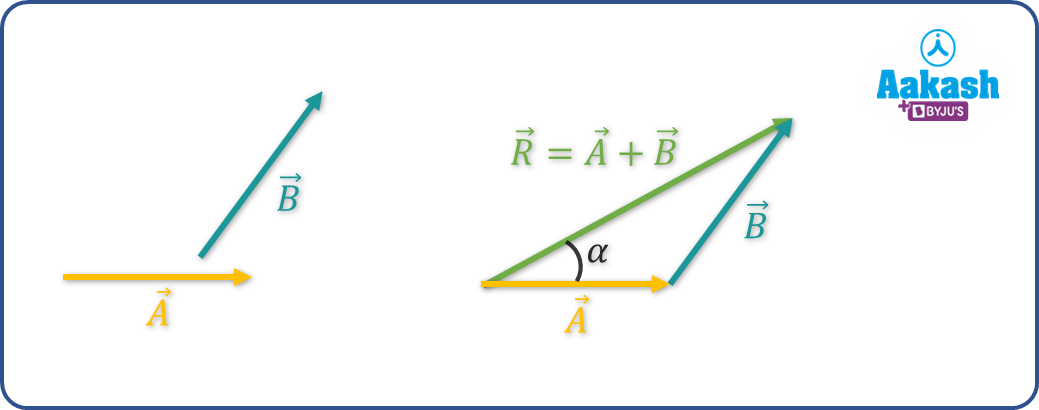If is the angle between vector A and B then magnitude of resultant R is given by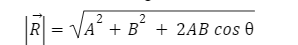And if is the angle of resultant from A then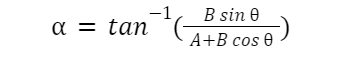## Parallelogram Law of Vector Addition

In the Parallelogram law of vector addition, When two vectors are represented by the two adjacent sides of a parallelogram tail to tail, then the diagonal of the parallelogram will represent the resultant vector starting from the common point.

Suppose u and v are the two vectors, then resultant of these vectors R=A+B.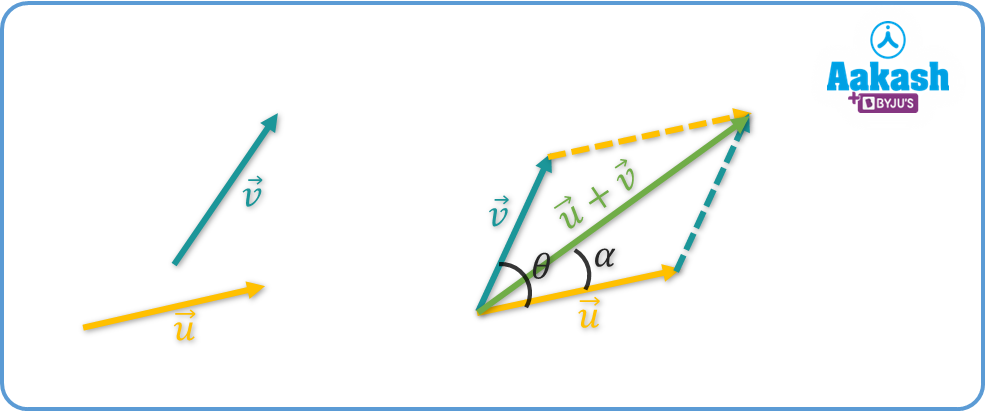If is the angle between vector u and v then magnitude of resultant R is given by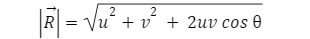And if is the angle of resultant from u then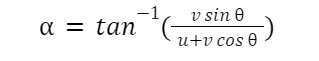## Polygon Law of Vector Addition

In Polygon law of vector addition, if a number of vectors is represented in magnitude and direction by the sides of a polygon having same no of side of vector, then their resultant is represented in magnitude and direction by the remaining side of the polygon taken in the opposite order.

From figure we can see if we have two vector a and b then resultan is represented by vector OB and if vectors are a, b and c then resultant is represented by vector OC and so on.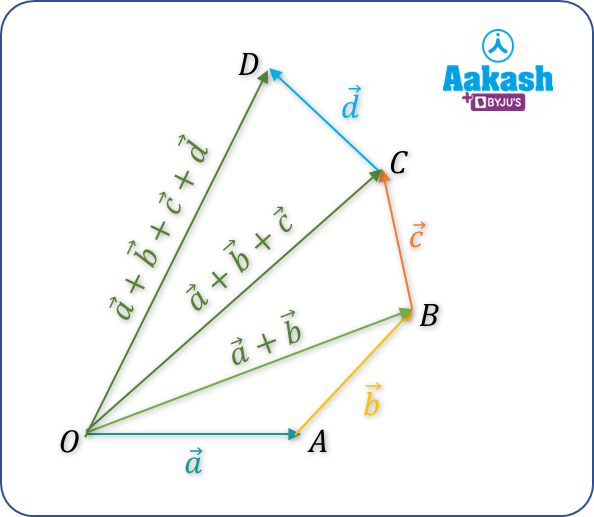• Commutative: It states the order of addition doesn't matter.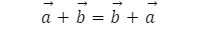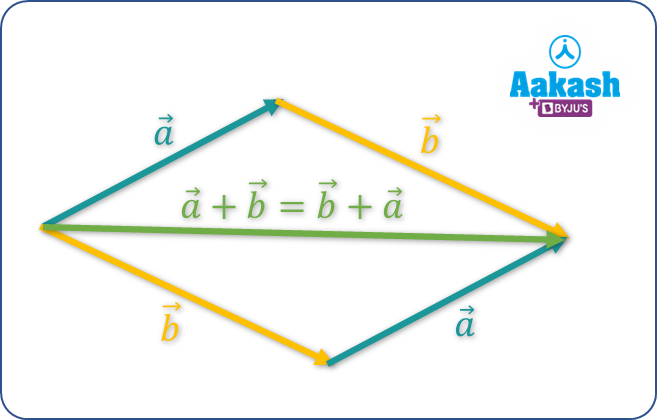• Associative: the sum of three vectors does not affected by which pair of vectors is added first: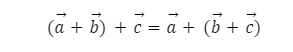• Distributive: Vector addition is distributive i.e.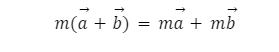• Additive Identity: If you add a null vector to any vector, the result will always remain the same.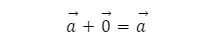## Practice Problems of Vector Addition

Question.1 Find the angle between A and B.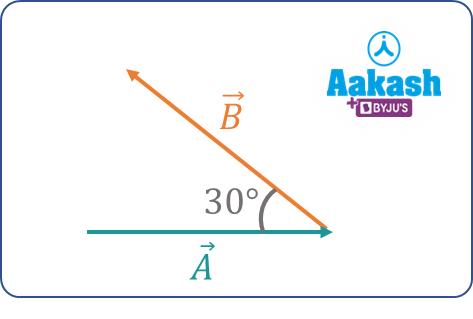Answer: After shifting vector A the diagram is shows as

So, the angle between two vector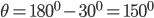Question.2  Vector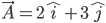and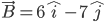find the resultant of two vectors R.Question.3 magnitude of A is 10 and B is 15 and angle between them is 600.find the magnitude of the resultant vector.

Answer: magnitude of resultantQuestion.3 if Magnitude of P is 5 and Q is 3 and angle between them is 600.find the angle of resultant vector with P.

Answer: Angle of resultantQuestion.1 What do you mean by vector addition?

Answer: Vector addition means putting two or more vectors together and finding the resultant.

Question.2  What is the Triangle Law of Addition of Vectors?

Answer: When two vectors are represented by the two sides of a triangle then the third side will represent the resultant vector.

Question.3  What is the commutative property of a vector?

Question.4  Can the addition of two vectors may have zero?

Answer: Yes, if the magnitude is equal and directions are opposite.

## NCERT Class 11 Physics Chapters

 Physical World Units and Measurements Motion in a Straight Line Motion in a Plane Laws of Motion Work Energy and Power Particles and Rotational Motion Gravitation Mechanical Properties of Solids Mechanical Properties in Liquids Thermal Properties of Matter Thermodynamics Kinetic Theory Oscillations WavesTalk to our expert
Resend OTP Timer =
By submitting up, I agree to receive all the Whatsapp communication on my registered number and Aakash terms and conditions and privacy policy